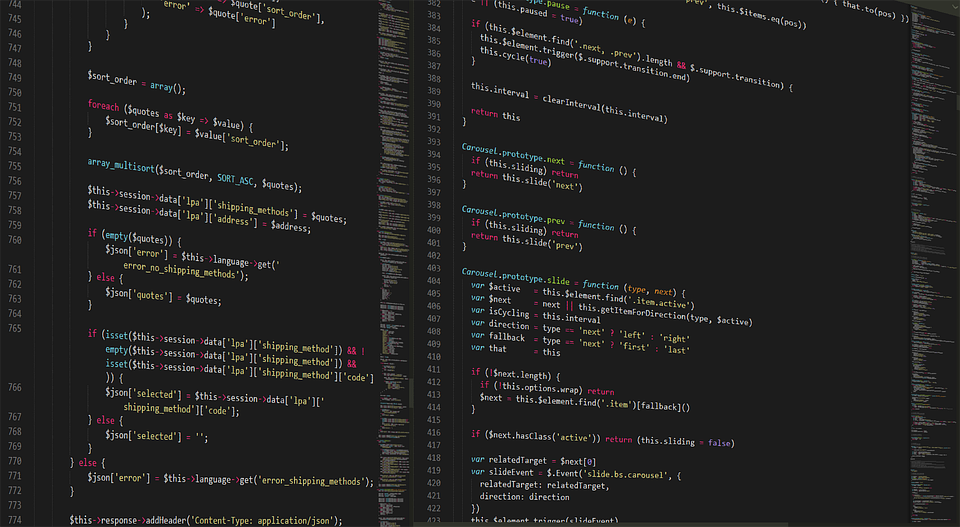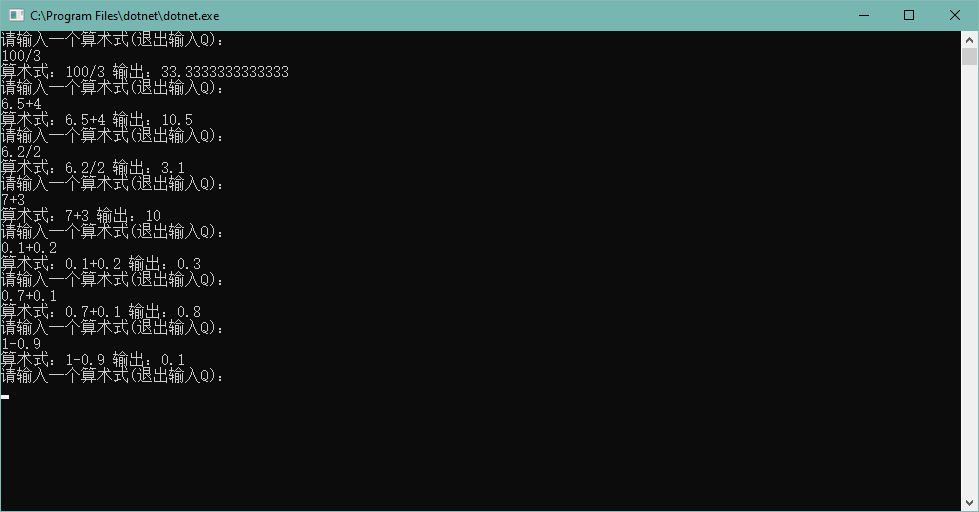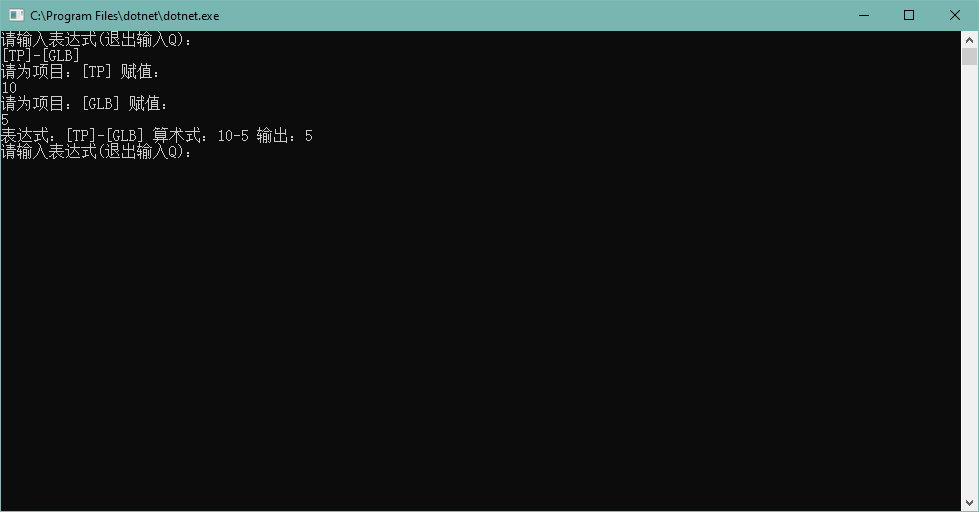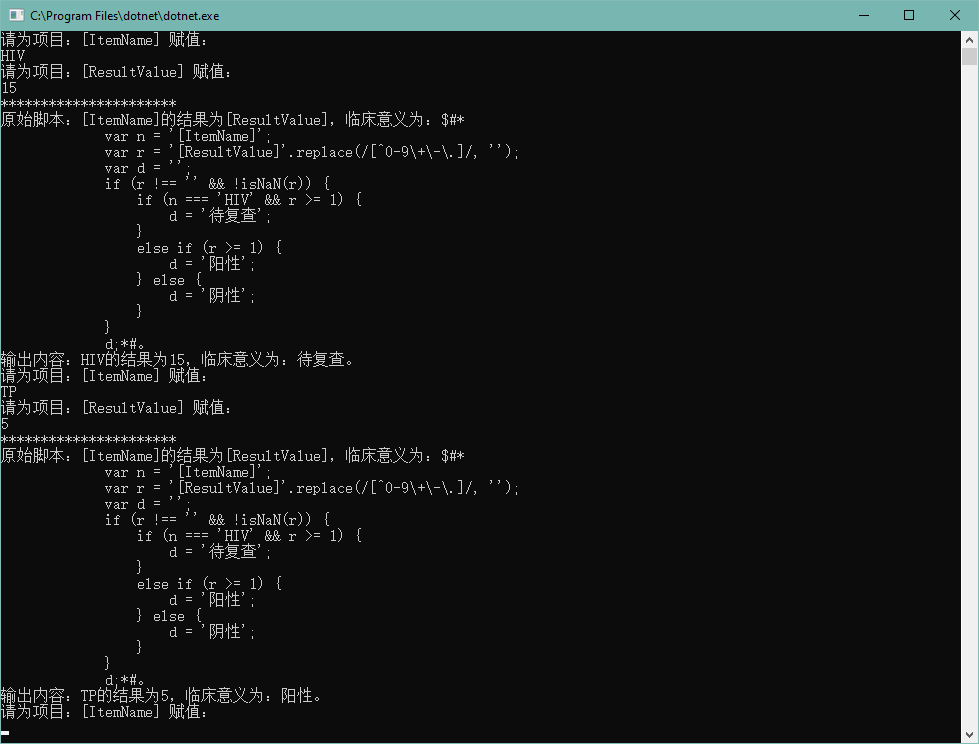# 利用Jint在C#中运行JS脚本并实现简单计算器

## 关于Jint

Jint是一个开源的JS脚本引擎，可以让我们在dotnet平台运行js代码，这使我们可以通过这一特性处理很多工作。

## Jint的用途

### 数学运算

1. 使用数据库拼接SQL语句；
2. 通过C#反射实现类似js的eval函数效果；

/// <summary>
/// 获取算术式结果
/// </summary>
/// <param name="input">原始算术式</param>
public static void Calculator(string input = null)
{
do
{
//算式为空提示输入算式
if (string.IsNullOrEmpty(input))
{
Console.WriteLine("请输入一个算术式(退出输入Q)：");
}

//非退出该方法
if (!string.Equals(input, "Q", StringComparison.OrdinalIgnoreCase))
{
try
{
//使用Jint执行算式
Engine engine = new Engine();
object result = engine.Execute(input).GetCompletionValue().ToObject();
Console.WriteLine($"算术式：{input} 输出：{result}"); } catch (Exception exc) { Console.WriteLine($"算术式：{input} 运算出现异常：{exc.Message}");
}

//赋值空让用户循环输入
input = null;
}
} while (!string.Equals(input, "Q", StringComparison.OrdinalIgnoreCase));
}/// <summary>
/// 获取表达式结果
/// </summary>
/// <param name="input">原始表达式</param>
public static void GetResultFromExpression(string input = null)
{
string oldInput;
Regex regex = new Regex(@"$([A-Za-z0-9]+)$");//表达式中项目由字母和数字组成
do
{
//表达式为空提示输入表达式
if (string.IsNullOrEmpty(input))
{
Console.WriteLine("请输入表达式(退出输入Q)：");
}

//用于输出原始表达式
oldInput = input;

//用于判断表达式项目是否已经替换
List<string> items = new List<string>();

//非退出该方法
if (!string.Equals(input, "Q", StringComparison.OrdinalIgnoreCase))
{
//匹配表达式中项目并替换
MatchCollection matches = regex.Matches(input);
foreach (Match match in matches)
{
//已经替换的跳过
if (items.Contains(match.Value))
{
continue;
}
Console.WriteLine($"请为项目：{match.Value} 赋值："); string item = Console.ReadLine(); input = input.Replace(match.Value, item); } try { //使用Jint执行表达式 Engine engine = new Engine(); object result = engine.Execute(input).GetCompletionValue().ToObject(); Console.WriteLine($"表达式：{oldInput} 算术式：{input} 输出：{result}");
}
catch (Exception exc)
{
Console.WriteLine($"表达式：{oldInput} 算术式：{input} 运算出现异常：{exc.Message}"); } //赋值空让用户循环输入 input = null; oldInput = null; } } while (!string.Equals(input, "Q", StringComparison.OrdinalIgnoreCase)); }  测试效果如下：### 自定义脚本 系统中某些位置，可能需要比较大的自由度去处理业务数据，例如文档的输出，我们能从数据库中读取到结果是数值型，但是实施人员希望我们能处理成文本型，并且设定的条件较多，自由度很大。 当然最直接的方案就是我们将这些集成到业务代码中，友好一点的设置一个参数表，由工程人员维护。但是当这里的业务逻辑复杂或者存在很多的不定因素，这里的维护更新就变得困难。 而这个时候，我们可以通过写一些js脚本，来处理复杂逻辑的问题，例如文档中我们配置了一下内容： [ItemName]的结果为[ResultValue]，临床意义为：[Descript]。 [ItemName]显示的内容是“HIV”时，[ResultValue]的值小于1判定[Descript]为“阴性”，否则为待复查。若[ItemName]不是“HIV”时，[ResultValue]的值小于1判定[Descript]为“阴性”，否则为阳性。如果[ResultValue]中存在非数字部分，则只取数字部分内容。 这里如果用业务代码或者参数来写，可能复杂一些，因为像这样的业务逻辑还有很多，而且可能随时需要调整，但是我们可以将以上的内容稍稍进行调整，我们定义$#*[JS代码]*#来包括一段代码，将以上的内容进行调整：

[ItemName]的结果为[ResultValue]，临床意义为：$#* var n = '[ItemName]'; var r = '[ResultValue]'.replace(/[^0-9\+\-\.]/, ''); var d = ''; if (r !== '' && !isNaN(r)) { if (n === 'HIV' && r >= 1) { d = '待复查'; } else if (r >= 1) { d = '阳性'; } else { d = '阴性'; } } d;*#。  那么我们写一个测试用例实现以下对以上内容的解析： /// <summary> /// 执行自定义脚本获取内容 /// </summary> /// <param name="input">脚本</param> public static void ExecuteCustomScript(string input = null) { string oldInput; Regex regex1 = new Regex(@"$([A-Za-z0-9]+)$");//脚本中项目由字母和数字组成 Regex regex2 = new Regex(@"\$#\*(.+)\*#", RegexOptions.Singleline);//匹配自定义脚本
do
{
//脚本为空提示输入
if (string.IsNullOrEmpty(input))
{
Console.WriteLine("请输入脚本(退出输入Q)：");
}

//用于输出原始脚本
oldInput = input;

//用于判断脚本项目是否已经替换
List<string> items = new List<string>();

//用于判断脚本内容是否已经替换
List<string> scripts = new List<string>();

//非退出该方法
if (!string.Equals(input, "Q", StringComparison.OrdinalIgnoreCase))
{
//匹配脚本中项目并替换
{
MatchCollection matches = regex1.Matches(input);
foreach (Match match in matches)
{
//已经替换的跳过
if (items.Contains(match.Value))
{
continue;
}
Console.WriteLine($"请为项目：{match.Value} 赋值："); string item = Console.ReadLine(); input = input.Replace(match.Value, item); } } //匹配内容中自定义脚本并执行替换 { MatchCollection matches = regex2.Matches(input); foreach (Match match in matches) { //已经替换的跳过 if (scripts.Contains(match.Value)) { continue; } scripts.Add(match.Value); try { //使用Jint执行内容中自定义脚本 Engine engine = new Engine(); object result = engine.Execute(match.Groups.Value).GetCompletionValue().ToObject(); input = input.Replace(match.Value, result.ToString()); } catch (Exception exc) { Console.WriteLine($"脚本：{match.Groups.Value}\r\n执行出现异常：{exc.Message}");
}
}
}

Console.WriteLine($"**********************\r\n原始脚本：{oldInput}\r\n输出内容：{input}"); //还原公式让用户重新赋值 input = oldInput; } } while (!string.Equals(input, "Q", StringComparison.OrdinalIgnoreCase)); }  调用： JintTest.ExecuteCustomScript(@"[ItemName]的结果为[ResultValue]，临床意义为：$#*
var n = '[ItemName]';
var r = '[ResultValue]'.replace(/[^0-9\+\-\.]/, '');
var d = '';
if (r !== '' && !isNaN(r)) {
if (n === 'HIV' && r >= 1) {
d = '待复查';
}
else if (r >= 1) {
d = '阳性';
} else {
d = '阴性';
}
}
d;*#。");### 评论

###### Your browser is out-of-date!

Update your browser to view this website correctly. Update my browser now

×